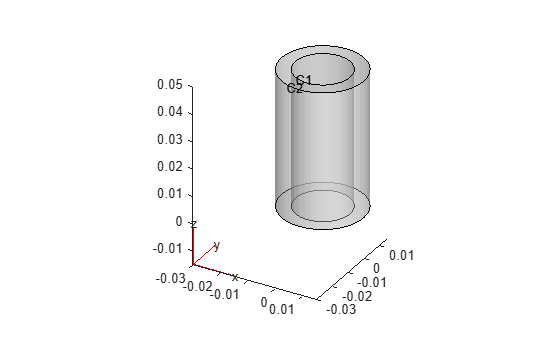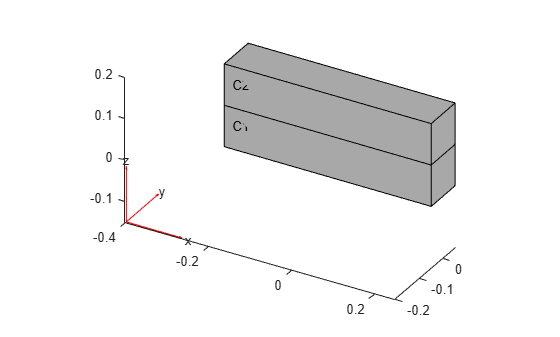Since R2023a

## Description

A `cellLoad` object contains a description of a load on a cell of a geometry. An `femodel` object contains an array of `cellLoad` objects in its `CellLoad` property.

## Creation

### Syntax

``model.CellLoad(CellID) = cellLoad(Name=Value)``
``model.CellLoad = cellLoad(Name=Value)``

### Description

example

````model.CellLoad(CellID) = cellLoad(Name=Value)` creates a `cellLoad` object and sets properties using one or more name-value arguments. This syntax assigns the specified structural, thermal, or electromagnetic load to the specified cells of the geometry stored in the `femodel` object `model`. For example, `model.CellLoad(1) = cellLoad(Gravity=[0 0 -9.8])` specifies the gravity load on cell 1.```

example

````model.CellLoad = cellLoad(Name=Value)` assigns the specified load to the entire geometry. For example, ```model.CellLoad = cellLoad(Gravity=[0 0 -9.8])``` specifies the gravity load on all cells of the geometry.```

### Input Arguments

expand all

Cell IDs, specified as a vector of positive integers. Find the cell IDs using `pdegplot` with the `CellLabels` value set to `"on"`.

Data Types: `double`

## Properties

expand all

Acceleration due to gravity, specified as a vector of three elements for a 3-D model.

Data Types: `double`

Angular velocity for modeling centrifugal loading in an axisymmetric model, specified as a positive number.

Data Types: `double`

Thermal load, specified as a real number, `SteadyStateThermalResults` object, or `TransientThermalResults` object. This property must be specified in units consistent with those of the geometry and material properties. For `TransientThermalResults`, you can access results for a particular time-step by using the `filterByIndex` function.

Tip

If you specify a thermal load, you must also specify a reference temperature using `model.ReferenceTemperature`. For details, see the description of the `ReferenceTemperature` property in `femodel`.

Heat source term, specified as a real number or function handle. Use a function handle to specify an internal heat source that depends on space, time, or temperature. For details, see Nonconstant Parameters of Finite Element Model.

Data Types: `double` | `function_handle`

Charge density, specified as a real number or function handle. Use a function handle to specify a charge density that depends on the coordinates. For details, see Nonconstant Parameters of Finite Element Model.

Data Types: `double` | `function_handle`

Current density, specified as a real number, vector, function handle, or a `ConductionResults` object. Use a function handle to specify a nonconstant current density. For details, see Nonconstant Parameters of Finite Element Model.

For magnetostatic analysis, the current density must be a vector of three elements, a `ConductionResults` object, or a function handle.

For harmonic analysis with an electric field type, the toolbox multiplies the specified current density by `-i` and by frequency. The current density must be a vector of three elements or a function handle that depends on the coordinates.

For harmonic analysis with a magnetic field type, the toolbox uses the curl of the specified current density. The current density must be a vector of three elements or a function handle that depends on the coordinates for a 3-D model.

Data Types: `double` | `function_handle`

Magnetization, specified as a vector of three elements or a function handle. Use a function handle to specify a magnetization that depends on the coordinates. For details, see Nonconstant Parameters of Finite Element Model.

Data Types: `double` | `function_handle`

## Examples

collapse all

Specify magnetization on a cell for an `femodel` object representing a magnetostatic problem.

Create and plot a geometry that consists of two nested cylinders.

```gm = multicylinder([0.01,0.015],0.05); pdegplot(gm,CellLabels="on",FaceAlpha=0.4);```Create an `femodel` object for solving a magnetostatic problem, and assign the geometry to the model.

```model = femodel(AnalysisType="magnetostatic", ... Geometry=gm);```

To make the inner cylinder represent a permanent magnet, specify the uniform magnetization in the positive x-direction.

```model.CellLoad(1) = cellLoad(Magnetization=[1 0 0]); model.CellLoad```
```ans = 1x2 cellLoad array Properties for analysis type: magnetostatic Index CurrentDensity Magnetization 1 [] [1 0 0] 2 [] [] Show all properties ```

Specify the gravity load for an `femodel` object representing a static structural problem.

Create and plot a bimetallic beam geometry.

```L = 0.5; W = 0.1; H = 0.1; gm = multicuboid(L,W,[H,H],Zoffset=[0,H]); pdegplot(gm,CellLabels="on");```Create an `femodel` object for solving a static structural problem, and assign the geometry to the model.

```model = femodel(AnalysisType="structuralStatic", ... Geometry=gm);```

Specify the gravity load on the entire beam.

```model.CellLoad = cellLoad(Gravity=[0 0 -9.8]); model.CellLoad```
```ans = 1x2 cellLoad array Properties for analysis type: structuralStatic Index Gravity AngularVelocity Temperature 1 [0 0 -9.8000] [] [] 2 [0 0 -9.8000] [] [] Show all properties ```

## Version History

Introduced in R2023a